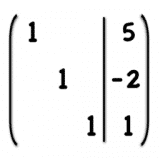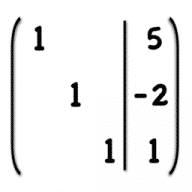Equation Systems
1.0

0.0

Equation Systems# Equation Systems1.0

15 August 2014

Solve linear systems of equations.

## Overview

Equation Systems is an app to solve linear systems of equations using Gauss-Jordan elimination. In the Gauss-Jordan algorithm, elementary row operations are applied to the system of equations until the matrix is in reduced-row echelon form, from which the solution can be read directly.

Main features:

• Unlimited number of variables and equations
• Representation of the individual matrices
• Representation of the individual row operations
• Entering a text to the equation system
This app is for anyone who deals with linear equation systems, especially those who want to learn the steps to solve them. Useful for school and study!

#### Join over 500,000 subscribers.

Subscribe for our newsletter with best Mac offers from MacUpdate.

How would you rate Equation Systems app?